# Skid friction

Find the smallest coefficient of skid friction between the car tires and the road so that the car can drive at a 200 m radius at 108 km/h and does not skid.

k =  2.18

### Step-by-step explanation: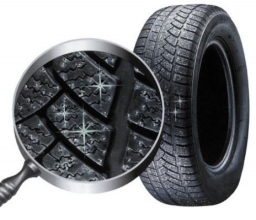Did you find an error or inaccuracy? Feel free to write us. Thank you!Tips to related online calculators

## Related math problems and questions:

• Friction coefficientWhat is the weight of a car when it moves on a horizontal road at a speed of v = 50 km/h at engine power P = 7 kW? The friction coefficient is 0.07
• The bridge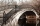A vehicle weighing 5,800 kg passes 41 km/h on an arched bridge with a radius of curvature of 62 m. What force is pushing the car onto the bridge as it passes through the center? What is the maximum speed it can cross over the center of the bridge so that
• Accelerated motion - mechanicsThe delivery truck with a total weight of 3.6 t accelerates from 76km/h to 130km/h in the 0.286 km long way. How much was the force needed to achieve this acceleration?
• G forcesCalculate deceleration of car (as multiple of gravitational acceleration g = 9.81 m/s2) occurs when a vehicle in a frontal collision slows down uniformly from a speed 111 km/h to 0 km/h in 1.2 meters trajectory.
• ICE train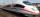German runways test a new ICE train between Munich and Berlin. The train runs to Berlin at a slow speed of 100 km/h. Back from Berlin goes faster. How quickly did the train have to go on a return trip so that the total average train speed for both journey
• An accelerationThe car goes on a straight road at a speed of 72 km/h. At some point, the driver starts to brake and stops the car in 5 seconds. Find: (a) the acceleration during braking (b) the distance traveled during braking.
• Deceleration of carThe car has a speed of 60 km/h and after 100 m journey speed of 40 km/h. What is the deceleration of a car if we assume that its movement is constantly slowed down?
• AccelerationThe car accelerates at a rate of 0.5 m/s2. How long travels 400 meters, and what will be its speed?
• Acceleration 2if a car traveling at a velocity of 80 m/s/south accelerated to a velocity of 100 m/s east in 5 seconds, what is the cars acceleration? using Pythagorean theorem
• BrakesThe braking efficiency of a passenger car is required to stop at 12.5 m at an initial speed of 40 km/h. What is the acceleration braking by brakes?
• Cars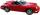Cars started at 7:00 AM. From point A car run at 40 km per hour, from B car run 70 km per hour. Between A and B is a 250 km long road. When and where will meet (measured from point A)?
• The carThe car weight 1280 kg, increased its speed from 7.3 m/s to 63 km/h on a track of 37.2 m. What force did the car engine have to exert?
• The projectileThe projectile was fired horizontally from a height of h = 25 meters above the ground at a speed of v0 = 250 m/s. Find the range and flight time of the projectile.
• CarThe driver of a car is to get to 608 km distant city. From atlas found that 162 km will have to pass through the cities at an average speed 48 km/h. The remainder of the journey passes outside the cities at average speed 116 km/h. Calculate how many hours
• Car overtaking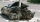A passenger car travels at a speed of 30 m/s, and before it travels freight truck that drives at a constant speed of 10 m/s. Within 30 meters of the truck, the driver of the car finds out that the truck can not overtake. That's why it starts braking with
• Two cars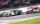Car A1 goes at average speed of 106 km/h and the second car A2 goes at 103 km/h. How many second will it take car A1 to circulate car A2? Assume that both cars are 5 meters long and the safety gap between cars is 1.5 meters.
• Brakes of a carFor the brakes of a passenger car to be effective, it is prescribed that a car be moving on a horizontal road at a speed of 40 km. A car must stop on the track 15.4 m. What is the deceleration of the car?